### Examples of Special Features

 Power Analysis using StudySize 3.0. Bioequivalence trial with interim analysis A new formulation of a drug has been developed. A two-way crossover study is planned to compare this new formulation with the existing formulation. The concentration of the active substance is measured over a 24-hour time interval and the area under the concentration curve (AUC) is calculated. The new formulation is considered bioequivalent to the old one if the ratio of the true mean AUC can be concluded to be within the interval 0.80 to 1.25. The null-hypothesis is that the true ratio is outside the interval. Bioequivalence is concluded if the null-hypothesis is rejected. The null-hypothesis is rejected at an upper significance level of 0.05 if the two one-sided tests for testing the ratio is less than 0.8 and greater than 1.25, respectively, both are rejected at the significance level 0.05 (two one-sided test situation). It can be shown that this is equivalent to a confidence interval for the true ratio, with confidence level 0.90 is entirely within the interval 0.8 to 1.25. The analysis for the two-way crossover design is performed using an ANOVA on the log-transformed AUC values. The study is planned to have a power of 0.80 to conclude bioequivalence if the true ratio is approximately 1.05 at the significance level 0.05. The expected residual standard deviation in the ANOVA (the within subject standard deviation) for the log-transformed AUC values is assumed to be in the range 0.15 to 0.25. Open the File menu and choose New Table. Open the Test Procedure menu and choose Bioequivalence test. Set the following options and click OK.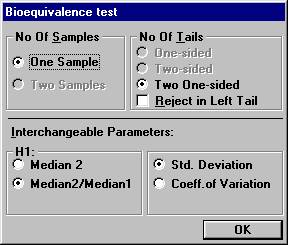Set the following values and click OK.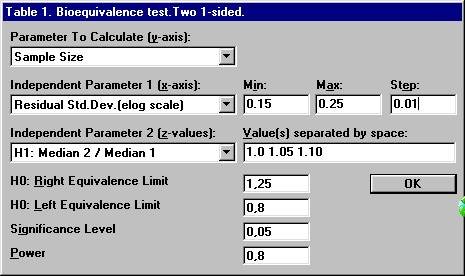This will create a table where the sample size is calculated for a range of values for the residual standard deviation and for three distinct values of the true AUC ratio, 1.0, 1.05 and 1.10. Note that the sample size is treated as a continuous parameter.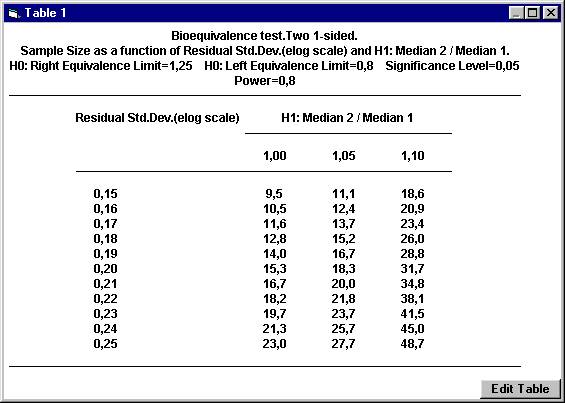The table shows the sample sizes needed if the true AUC ratio is 1.05 and the residual standard deviation is in the interval 0.15 to 0.25. It also shows how much smaller and larger the sample size has to be if the assumption about the true AUC ratio instead is set to 1.0 and 1.10. Since the study is planned to be a 2-period crossover study and we usually want the same number of patients in the two possible formulation sequences, the sample sizes should be rounded upwards to the nearest even integer. For example, with a ratio of 1.05 and a residual standard deviation of 0.20, the sample size 18.3 should be rounded upwards to 20. To calculate the power for 20 subject, Open the File menu and choose New Calculation. Open the Test Procedure menu and choose Bioequivalence test. The dialogbox with the retained values will show up. Click the OK button. Set the following values except for power and then click the Power button.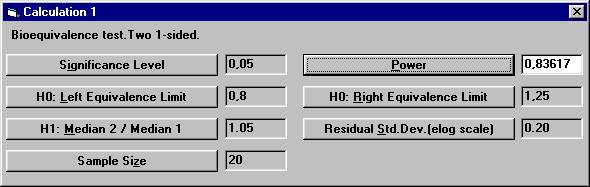There is now a suggestion to investigate if bioequivalence can be concluded before all 20 subjects have entered the study. Open the File Menu and choose New Monte Carlo Simulation.Open the Test Procedure menu and choose Bioequivalence test. Set the number of interim analyses to 1 in the dialogbox. The old parameter settings are retained. Click the OK button.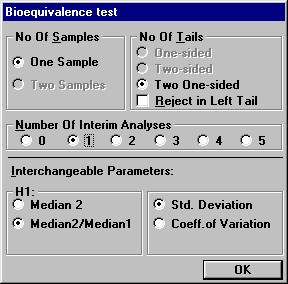A new dialog box will be shown. To compensate for two analyses, one has to choose the significance level at the interim analysis and the final analysis in such a way that the over-all significance level will be 0.05. There are many possibilities for such a design. Set e.g. the values as shown below and click OK.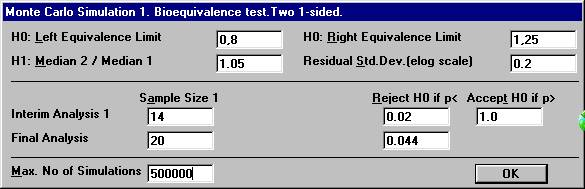If one prefers to use confidence intervals instead of the p-values, the corresponding confidence levels are 96% at the interim analysis, and 91.2% at the final analysis. Bioequivalence is concluded if the respective confidence interval is within the interval 0.80 to 1.25. The results are presented below after 500.000 Monte Carlo simulations.The significance level is controlled and approximately 0.05 and the power is 0.825. The average number of subjects when H1 is true is 0.4263 * 14 + (1 – 0.4263) * 20 = 17.4, a gain of 2 subjects compared to 20 but with somewhat lower power. However, there is a large chance (0.4263) that 14 subjects will suffice to show bioequivalence.